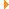C++ Async Framework (beta)Searching...
No Matches
concurrent::MutexSet< Key, Hash, Equal > Class Template Referencefinal

A dynamic set of mutexes. More...

`#include <userver/concurrent/mutex_set.hpp>`Inheritance diagram for concurrent::MutexSet< Key, Hash, Equal >:Collaboration diagram for concurrent::MutexSet< Key, Hash, Equal >:

## Public Member Functions

MutexSet (size_t ways=1, size_t way_size=1, const Hash &hash=Hash{}, const Equal &equal=Equal{})

ItemMutex< Key, Equal > GetMutexForKey (Key key)

## Detailed Description

template<typename Key = std::string, typename Hash = std::hash<Key>, typename Equal = std::equal_to<Key>>
class concurrent::MutexSet< Key, Hash, Equal >

A dynamic set of mutexes.

It can be used for separate critical sections for multiple keys when the key set is not known at compile time and may change in runtime.

Example:

auto m1 = ms.GetMutexForKey("1");
auto m2 = ms.GetMutexForKey("2");
auto m1_again = ms.GetMutexForKey("1");
{
std::unique_lock lock_first(m1);
std::unique_lock lock_second(m2);
// Mutex for key "1" already locked
EXPECT_FALSE(m1_again.try_lock());
}
// Mutex for key "1" is now unlocked
std::unique_lock lock(m1_again);

Definition at line 90 of file mutex_set.hpp.

## ◆ MutexSet()

template<typename Key , typename Hash , typename Equal >
 concurrent::MutexSet< Key, Hash, Equal >::MutexSet ( size_t ways = `1`, size_t way_size = `1`, const Hash & hash = `Hash{}`, const Equal & equal = `Equal{}` )
explicit

Definition at line 106 of file mutex_set.hpp.

## ◆ GetMutexForKey()

template<typename Key , typename Hash , typename Equal >
 ItemMutex< Key, Equal > concurrent::MutexSet< Key, Hash, Equal >::GetMutexForKey ( Key key )

Get the mutex-like object for a key. Coroutine-safe.

Note
the returned object holds a reference to MutexSet, so make sure that MutexSet is alive while you're working with ItemMutex.

Definition at line 112 of file mutex_set.hpp.

The documentation for this class was generated from the following file: